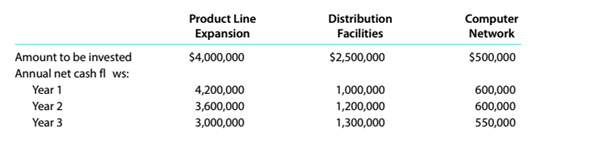Chapter 15, Problem 15.3.2P

Chapter
Section
Textbook Problem

Net present value method, present value index, and analysisDonahue Industries Inc. wishes to evaluate three capital investment projects by using thenet present value method. Relevant data related to the projects are summarized as follows:InstructionsDetermine a present value index for each project. Round to two decimal places.

To determine

Concept Introduction:

NPV:

Net present value (NPV) is the method to evaluate the project feasibility. This method calculates the present value of cash inflows and outflows, and then calculates the net present value of the investment. A project should be accepted if it has a positive NPV. The formula to calculate the NPV is as follows:

NPV = Present value of cash inflows  Present value of cash out flows

To Calculate:

The Present value Index for each project

Explanation

The Present value Index for each project is prepared as follows:

 Product Line Expansion Amount PVF (20%) PV A B =A*B Annual Net Cash Flows Year 1 $4,200,000 0.83333$ 3,500,000 Year 2 $3,600,000 0.69444$ 2,500,000 Year 3 $3,000,000 0.57870$ 1,736,111 Present Value of Cash Inflow (A) $7,736,111 Investment (B)$ 4,000,000 1.00000 $4,000,000 Present Value Index (A/B) 1.93 Distribution Facilities Amount PVF (20%) PV A B =A*B Annual Net Cash Flows Year 1$ 1,000,000 0.83333 $833,333 Year 2$ 1,200,000 0...

Still sussing out bartleby?

Check out a sample textbook solution.

See a sample solution

The Solution to Your Study Problems

Bartleby provides explanations to thousands of textbook problems written by our experts, many with advanced degrees!

Get Started

Who issues a credit memorandum and why?

College Accounting (Book Only): A Career Approach

How should the capital structure weights used to calculate the WACC be determined?

Fundamentals of Financial Management, Concise Edition (MindTap Course List)

What is the difference between condusions and recommendations in a report?

Essentials of Business Communication (MindTap Course List)

Discuss the following statement: If a firm has only independent projects, a constant WACC, and projects with no...

Fundamentals of Financial Management, Concise Edition (with Thomson ONE - Business School Edition, 1 term (6 months) Printed Access Card) (MindTap Course List)